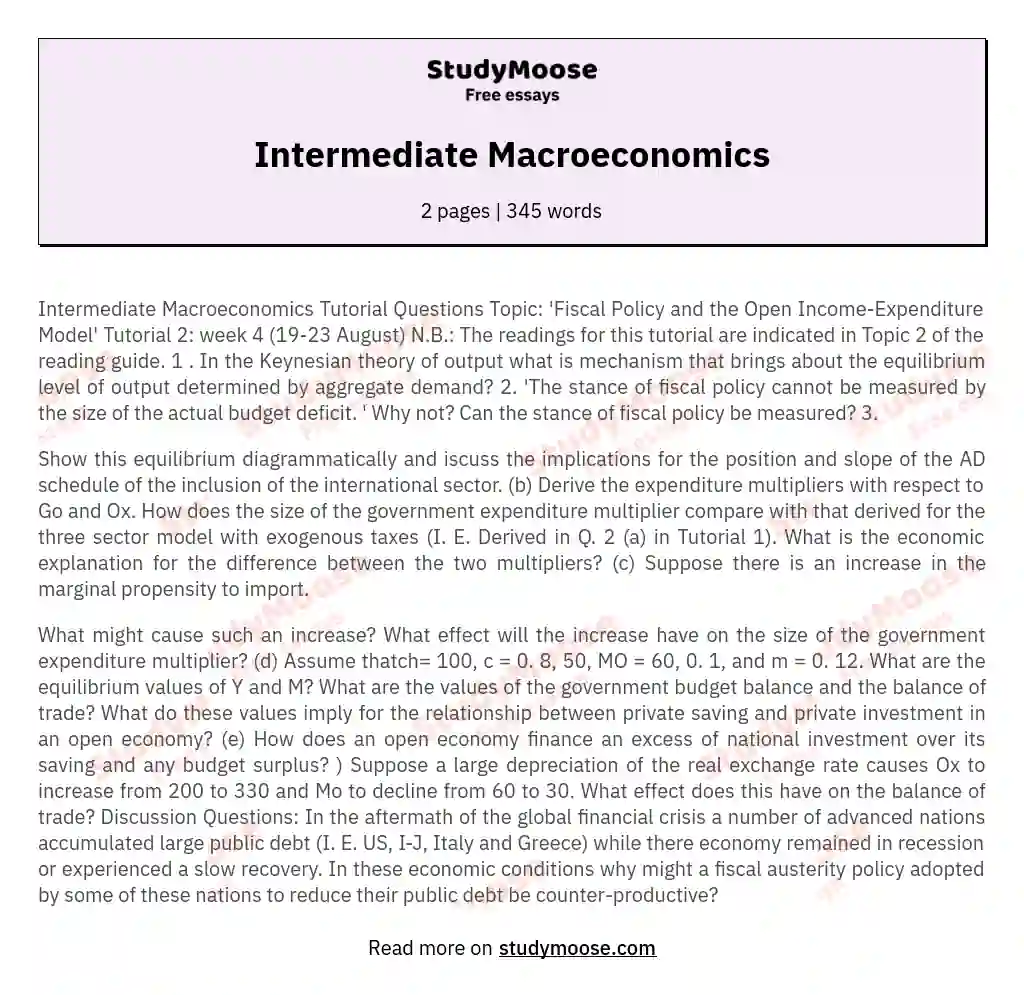# Intermediate Macroeconomics

Intermediate Macroeconomics Tutorial Questions Topic: 'Fiscal Policy and the Open Income-Expenditure Model' Tutorial 2: week 4 (19-23 August) N.B.: The readings for this tutorial are indicated in Topic 2 of the reading guide. 1 . In the Keynesian theory of output what is mechanism that brings about the equilibrium level of output determined by aggregate demand? 2. 'The stance of fiscal policy cannot be measured by the size of the actual budget deficit. ' Why not? Can the stance of fiscal policy be measured? 3.

Show this equilibrium diagrammatically and iscuss the implications for the position and slope of the AD schedule of the inclusion of the international sector.

(b) Derive the expenditure multipliers with respect to Go and Ox. How does the size of the government expenditure multiplier compare with that derived for the three sector model with exogenous taxes (I. E. Derived in Q. 2 (a) in Tutorial 1). What is the economic explanation for the difference between the two multipliers? (c) Suppose there is an increase in the marginal propensity to import.

Get quality help nowDoctor JenniferVerified writer

Proficient in: Economics5 (893)

“ Thank you so much for accepting my assignment the night before it was due. I look forward to working with you moving forward ”+84 relevant experts are online

What might cause such an increase? What effect will the increase have on the size of the government expenditure multiplier? (d) Assume thatch= 100, c = 0. 8, 50, MO = 60, 0. 1, and m = 0. 12. What are the equilibrium values of Y and M? What are the values of the government budget balance and the balance of trade? What do these values imply for the relationship between private saving and private investment in an open economy? (e) How does an open economy finance an excess of national investment over its saving and any budget surplus? ) Suppose a large depreciation of the real exchange rate causes Ox to increase from 200 to 330 and Mo to decline from 60 to 30.

Get to Know The Price Estimate For Your Paper
Topic
Number of pages
Email Invalid email

You won’t be charged yet!

What effect does this have on the balance of trade? Discussion Questions: In the aftermath of the global financial crisis a number of advanced nations accumulated large public debt (I. E. US, I-J, Italy and Greece) while there economy remained in recession or experienced a slow recovery. In these economic conditions why might a fiscal austerity policy adopted by some of these nations to reduce their public debt be counter-productive?